Brushless DC Motors Introduction for Next-Generation Missile Actuation Systems Outline

Munition systems are quickly becoming as cost constrained as other areas of engineering as costs rise and customers demand smaller and longer range products. In order to effectively drive and control these munitions in flight, a small control actuation system (CAS) makes small precise adjustments to place fins and adjust airflow over the body. Traditionally these systems were pneumatic or driven via a brushed dc motor with a gearbox, but modern advancements in motor drives including brushless dc motors (BLDC) have enabled smaller, lighter, cheaper, and more efficient CAS designs. However, this comes at the price of added system complexity to drive the three phases of the BLDC.

The added complexity comes from a number of sources. Firstly, while a traditional brushed dc motor only required a single H-bridge, a BLDC requires three separate pairs of MOSFETs to drive the phases. This adds a small amount of cost and requires additional land area on the PCB. When driving these MOSFETs, care must be taken to avoid current shoot through that could destroy the MOSFETs if both the top and bottom are enabled at the same time. Particular attention must be paid to the dead time inserted between the top and bottom drive lines for the pulse width modulation (PWM).

From a software perspective, a regular brushed motor could be controlled using a simple PID loop, while a BLDC will requires more advanced loops and commutation strategies—typically measuring the winding currents, phase voltages, rotor angle, and speed.

Construction of a BLDC

A BLDC is a collection of electromagnets on the stator with permanent magnets attached on the movable rotor. The motor can be either an inrunner (magnets on the inside of the coils) or an outrunner (magnets outside the coils). Figure 1 shows a BLDC inrunner and outrunner. In both cases, three phases of wires are wound (U, V, W or A, B, C) around teeth in the stator. These windings are energized in sequence to attract and repel the permanent magnets (in red/blue).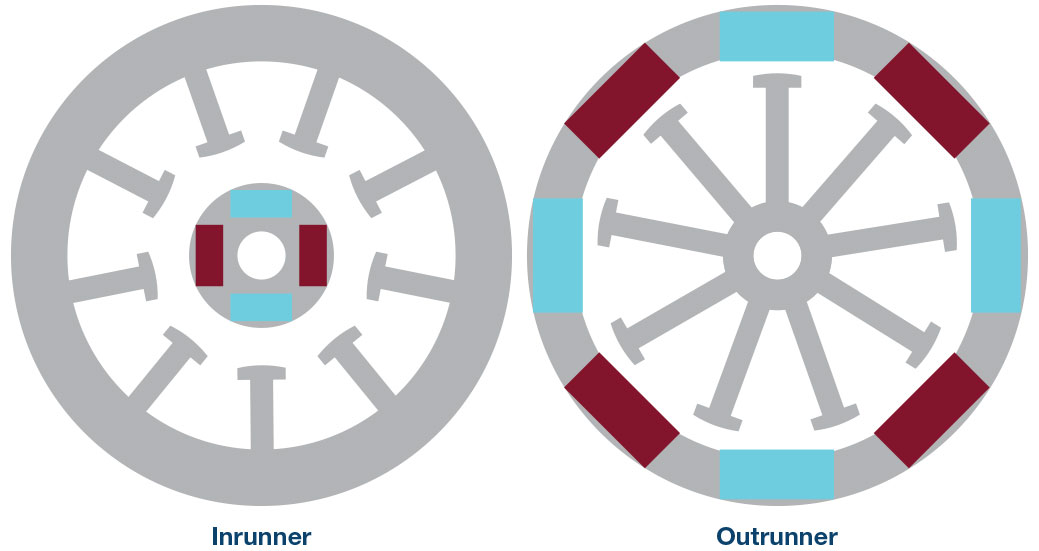Figure 1. Inrunner vs. outrunner.

A standard microprocessor or DSP does not have the current drive strength to adequately energize the coils directly and thus a power inverter stage consisting of MOSFETs (two per phase) is typically used to convert the PWM drive from the control interface to the required high voltage drive for the motor.

Normally, the three phase inverter uses six N-Channel MOSFETs (see the top portion of Figure 2), which produce the switching states shown in the bottom portion of Figure 2. There are several switching states that are not shown: 001, 010, 011, and 101. A 1 indicates one of the top three MOSFETs is enabled. These states map to a state space representation, as detailed by the six sectors in Figure 3. By turning the switches on and off, the voltages applied to the windings can reach a maximum of 2/3 × VDC. A natural extension of this strategy is to apply PWM to each pair of MOSFETs. By changing the duty cycle of the PWM waveforms, the generated voltage in the windings can create a wide range of voltages depending on the resolution of the PWM generation system.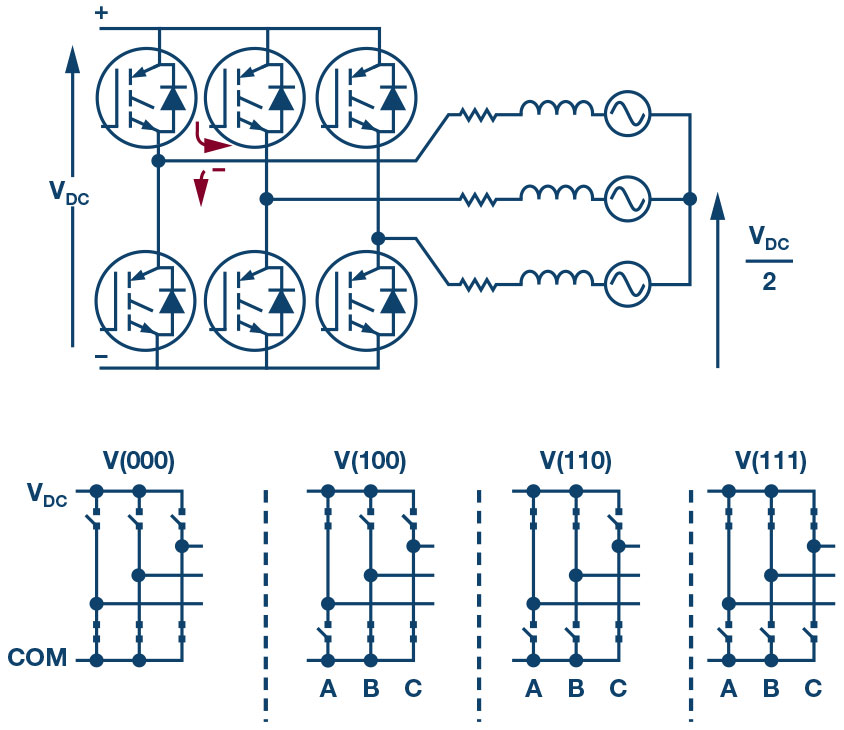Figure 2. Three phase inverter and switching states.Figure 3. Switch representations in state space and split into Sector I through Sector VI.

Without PWM, a very natural commutation strategy is to simply energize each pair of windings in series (that is, block commutation or six step commutation). For this strategy, one or two phases are pulled high while the remaining windings are driven low. By energizing the phases in sequence, the magnets on the rotor are pulled to each phase and the rotor begins to rotate. In order to determine what phases to energize, three Hall effect sensors are typically mounted 60 electrical degrees apart on the stator. The Hall effect sensors detect each of the rotor magnets and generate a 3-bit digital sequence, which is used to determine the next commutation region. While this strategy works well for low cost motor control systems, the strategy suffers from torque ripple at low speeds. Additionally, this torque ripple causes acoustic noise and introduces positional error if the motor is used for positioning/servo applications.1

Sinusoidal commutation works on the principle of aligning the stator currents with the stator flux in the BLDC. A BLDC moves based on the trapezoidal current through the windings. Each of these currents should be 120° out of phase. A permanent magnet synchronous motor (PMSM) is similar to a BLDC, but requires sinusoidal currents to drive. A block diagram of how to drive a PMSM is presented in Figure 4. Simple speed control is achieved by using a velocity sensor or by using an estimation based on a position shaft sensor and motor parameters. Sinusoidal drive works particularly well at low speeds, but falls apart at higher speeds as the electrical frequency of the required sine wave must also increase with speed. At higher speeds the motor requires higher torques, which introduce lag into the phase currents.2 To properly control at higher speeds, phase advances must typically be introduced to keep the torque and flux vectors properly aligned.Figure 4. Sinusoidal drive of a PMSM.

To overcome this problem, a more advanced control scheme called field-oriented control (FOC) can be implemented. As with other commutation strategies, FOC can be implemented sensorless with estimations of position and speed based on the back-EMF generated by the windings, or sensored with position and current sensors. The basis of FOC is the control of the torque and flux vectors going into the windings of the motor. The generation of these vectors comes from the desired speed input to the motor.

Using the electrical and mechanical constants of the motor (the moment of inertia, friction coefficient, inductance and resistance of the stator windings, and back-EMF constant), the PI loops from Figure 5 transform the desired speed into dc quantities. To control the electrical cycle of the motor, these quantities must be transformed into the reference frame of the rotor (Figure 6) to produce the Vα and Vβ using the Park transform. The next step in FOC is to convert Vα and Vβ to PWM values sent to the PWM inverter unit. Typically, either a sinusoidal modulation scheme (SPWM) or a space vector modulation scheme (SVPWM or SVM) is used for this process.Figure 5. FOC block diagram.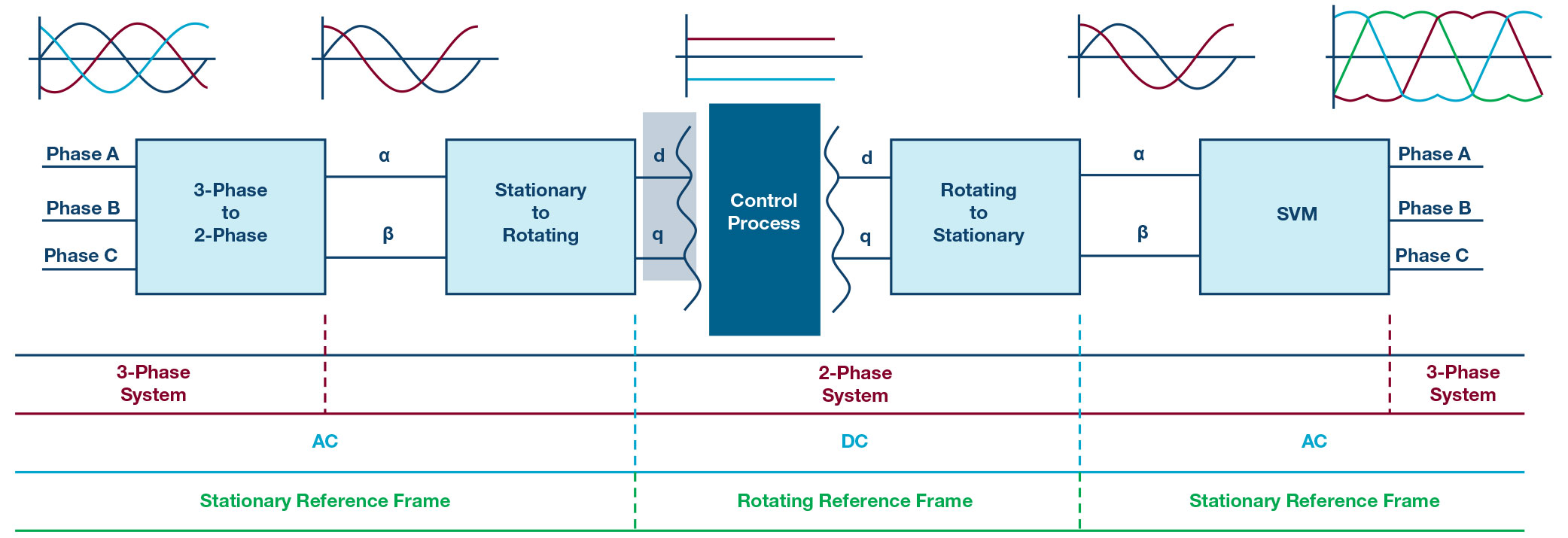Figure 6. Transformation of control parameters in FOC.

As mentioned previously, by controlling the switching of the MOSFETs within the power inverter, a space vector representation can be created, as in Figure 3. The space between adjacent unit vectors is coded to produce a sector between 1 and 6 to correspond to the six switching sectors of the electrical cycle of commutation. A close-up of Sector 1 from Figure 3 is shown in Figure 7. The voltage vector VREF is composed of the voltage vectors Vα and Vβ, and the angle θ is the inverse tangent of Vd divided by Vq.3 Figure 7 shows that the VREF can be derived by using two adjacent unit vectors (V1 and V2) and spending a particular amount time in each state (corresponding to a duty cycle). This duty cycle can be calculated by using equations similar to those derived from the vector math (see Figure 8).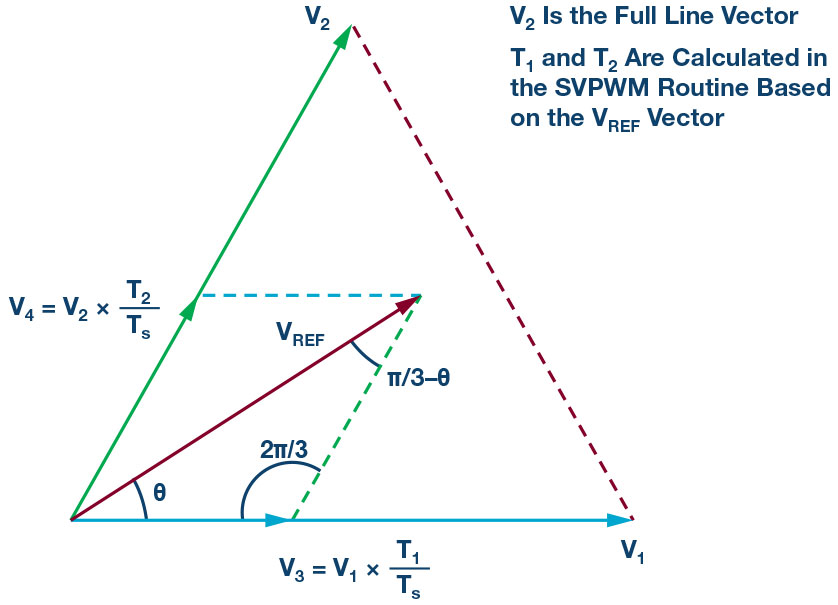Figure 7. Sector 1 of state space.

U, V, W Vector Equation CalculationFigure 8. Sector times from reference vectors.

From the equations in Figure 7, the PWM time can be found by using a normalized time of 1.0 (equal to a full 100% duty cycle) and subtracting the Tn and Tn+1. The sector can be determined through additional calculations, as shown in Figure 9.

Once the duty cycles are calculated and sent to the PWM module of the controller, open-loop control using FOC has been achieved. Now feedback must be integrated to achieve closed loop control. As Figure 4 shows, the three windings’ currents are measured and transformed using the inverse Clarke and inverse Park transforms. In order to measure these currents, several different strategies can be used: shunt sensing in line with each phase winding, a single low end shunt between the bottom three MOSFETs and ground, phase shunts beneath each MOSFET to ground, or high side shunts between each top MOSFET and the VDC. If the design is cost constrained, the method using two shunts in line with the windings provides a good measurement as this provides a straightforward way of measuring two winding currents directly. The third current can be calculated by using Kirchhoff’s current law and a sum of 0. An additional benefit is that the current can be measured at any moment, rather than only when the bottom or top MOSFETs are enabled. After measuring these currents, they should be normalized to the range of [–1, +1] using the max current measurable by the shunt system.

For position and speed sensing, either encoders (relative or absolute), Hall effect sensors, resolvers, or magnetic angular sensors can be used. However, depending on the resolution of the sensor, additional estimation schemes of the position and velocity may be necessary. Regardless of the technology, the angle measured must be converted to the electrical angle to synchronize the commutation with the actual rotor position and enable the rotor transforms. The angular speed must also be known, but this is typically kept in the mechanical domain to match the desired input speed.

With the actual position and speed of the rotor known, the inverse/reverse Park and Clarke transforms can be performed to convert the phase currents from the stationary stator reference frame to the rotating reference frame in the d, q reference frame. The PI loops for the resulting current and speed error terms create error vectors that are then fed back into the forward Park and Clarke transforms and the control process can repeat.

So how quickly should this process repeat? The answer varies depending on the motor characteristics. The PWM frequency is usually chosen to be outside the audible range of hearing (15 kHz to 30 kHz) such that the motor doesn’t audibly resonate. FOC and the required control loops are then implemented within the PWM interrupt service routine, such that new values for the PWM are available for the next PWM cycle. This places tight timing restrictions on the FOC routine as any time spent servicing the PWM interrupt is time not spent servicing other aspects of the control processor (such as serial interfaces to PC-based programs). With a PWM frequency of 30 kHz, each PWM ISR is only 33.3 µs. So every microsecond matters! Care must be taken to minimize computational overhead for sine and cosine and other floating-point computations. Generally, it is preferred to keep the FOC routine to under 50% of the available PWM ISR time, so the processor can service other peripherals such as the UART to service less important tasks such as changing desired speeds or setting a new position.

Selected Components

Given the already complex nature of implementing FOC, a careful selection of parts can help to minimize additional system integration challenges. Analog Devices offers a number of parts for motor control signal chains. These parts include gate drivers, absolute angle and Hall effect sensors, current sensors, and isolation products.

A simple block diagram of components for a motor control signal chain can be seen in Figure 10. At a high level, the BLDC shaft position and speed are sensed using the ADA4571 AMR angle sensor and AD22151 magnetic field transducer. The phase winding currents are measured using inline shunt resistors and the AD8418 current sense amplifiers remove the PWM common-mode voltage. The LTC2345-18 8× 18-bit ADC converts the 6 analog voltage from the sensors to the digital realm for the microcontroller. The microcontroller uses these signals to calculate the PWM duty cycle, which is sent to the hardware timers. The LT1158 MOSFET drivers act as gate drivers for the six MOSFETs of the power inverter.Figure 10. Block diagram of motor control signal chain.

The LT1158 is an integrated half bridge N-Channel MOSFET driver. While the supply can range from 5 V to 30 V dc, the input PWM waveform logic can accept a TTL or CMOS level. Additionally, a single PWM input is converted to the high and low MOSFET drive signals while the chip automatically inserts an adaptive dead time. This means that the PWM frequency can change dynamically and dead time is automatically inserted to protect the MOSFETs from current shoot-through without requiring changes to PWM timer code or registers.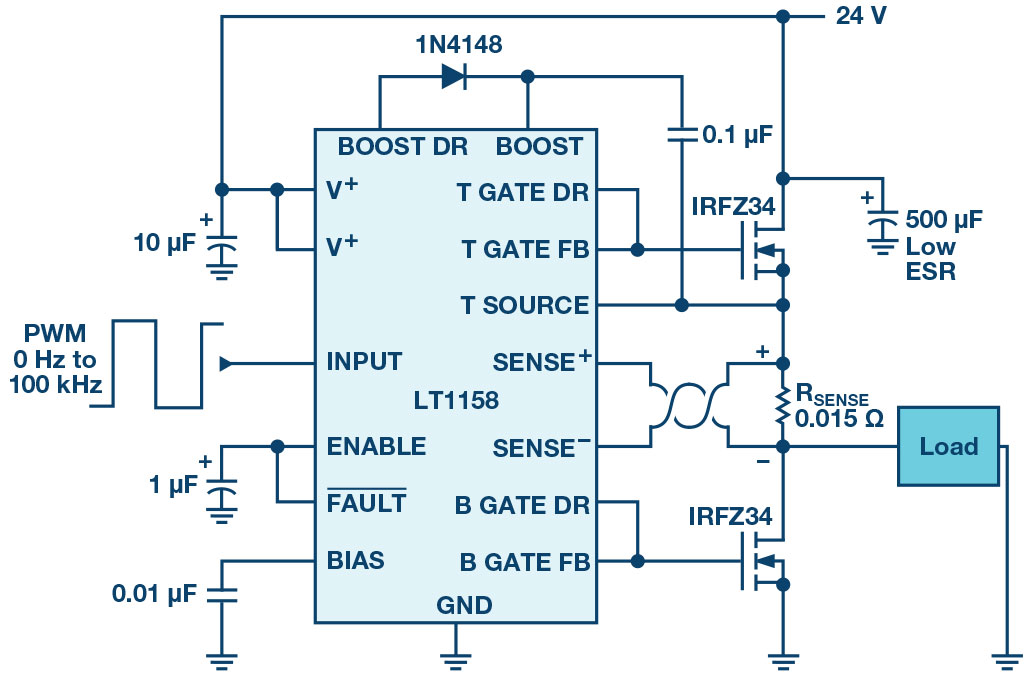Figure 11. LT1158 dual N-channel MOSFET driver.

The ADA4571 integrated anisotropic magnetoresistive (AMR) sensor is capable of measuring 180° of rotation to within 0.5°. This sensor runs from a single 2.7 V to 5 V supply and consumes only 7 mA with temperature compensation enabled. The output of this sensor is two analog sine waves (VSIN, VCOS) centered around 2.5 V (5 V supply). Once the voltages of VSIN and VCOS are digitized, they can be converted to an angle via the simple formula:

In order to measure 360° of absolute rotation, the ADA4571 can be combined with a linear output magnetic field (Hall effect) sensor such as the AD22151. The AD22151 is designed for single 5 V supply operation and outputs a voltage proportional linear to the magnetic field applied perpendicularly to the package. During normal operation the device draws a maximum of 10 mA and can detect bipolar or unipolar fields with varying amounts of gain. The benefit of this sensor is the analog output voltage, which can be easily added to a system already measuring analog quantities such as current sensor outputs or additional analog angle sensors. By placing the AD22151 perpendicular to the ADA4571, the outputs can be fused together in software to allow for sensing of 360° of motion of a shaft mounted diametric magnet.

Along with the angle sensors, sensored FOC requires precise measurement of the phase currents through a BLDC. The AD8418 is a bidirectional zero-drift current sense amplifier well suited for the task. This external shunt amplifier works with a gain of 20 V/V across temperature with a common-mode rejection range of –2 V to +70 V. The amplifier also senses bidirectional currents through the shunt, which is particularly useful when measuring phase currents for BLDCs. The part is designed to work with a supply voltage VS between 2.7 V and 5 V, with the analog output voltage centered around the VS/2. If the supply is chosen for 5 V, the output is centered around 2.5 V, as with the ADA4571.

With analog output sensors, the results must be converted to the digital domain. While a multitude of ADCs exist, the LTC2345 is particularly applicable for motor control due to 8 simultaneously sampling channels. Sampling is synchronized to a single rising edge on the convert line. Phase currents and the absolute angle sensor outputs can then be synchronized to the same time instant during center aligned PWM. The single 5 V supply operation simplifies power supply design, while still drawing less than 20 mA. A separate digital logic output voltage allows the LTC2345 to interface to lower voltage microcontrollers, processors, or FPGAs. Due to the flexible nature of the analog input ranges, the 2.5 V offset of the position and current sensors can automatically be removed in hardware by using the (IN–) analog input channels. Data can be clocked out of the LTC2345 using the SDO outputs at various clock rates depending on the required sampling throughput.Figure 14. LTC2345 block diagram.

Conclusions

With the increasing prevalence of BLDCs in control actuation systems, more advanced algorithms, sensors, and drive circuitry are required. Sinusoidal and FOC are two commutation strategies that afford precise control of a BLDC. Both strategies require precise measurement of the rotor angle of the BLDC, which can be difficult without the right components. However, the ADA4571 and AD22151 simplify this measurement. The LT1158 simplifies PWM drive lines for the three phases for a BLDC by reducing PWM lines and removing calculations of dead time. The AD8418 simplifies winding current and the LTC2345 easily digitizes and synchronizes multiple analog sensor outputs. These parts represent a small portion of the Analog Devices portfolio for motor control applications. For more information and design help, visit Analog Devices online.

Ссылки

2Danielle Collins. “FAQ: What Is Sinusoidal Commutation for DC Motors?” Motion Control Tips.

3Jin-Woo Jung. “Project #2 Space Vector PWM Inverter.” Ohio State University, February 2005.